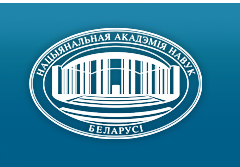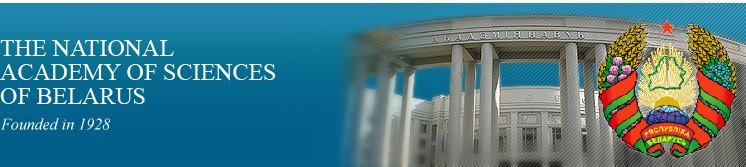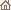Home News About Academy Academy Activities Academy Membership Organizations Publications Conferences Contacts Site Map

# Proc. of the Academy of Sciences of Belarus, Ser. Phys.-Math. Sci., 1997, No.1/ Publications / Scientific Journals

Proc. of the Academy of Sciences of Belarus, Ser. Phys.-Math. Sci., 1997, No.1Proceedings of the Academy of Sciences of Belarus (Vestsi Akademii Navuk Belarusi) SERIES OF PHYSICAL-MATHEMATICAL SCIENCESPublished by The Belaruskaya Navuka Publishing House, Minsk, Republic of Belarus

### Number 1, 1997## CONTENTS

### MATHEMATICS

Petrov V.A.
Diagram method based evaluation of functional integrals on the Poisson measure. pp. 5--8

Summary: An approximate formula for functional integrals on the Poisson measure is constructed. This formula takes consideration the contributions of special form Feynman diagrams.

Gaiduk S.I.
Problem about impact caused traversal oscillations of an elastoviscous beam with the supported ends. pp. 9--14

Summary: Solvability of mixed problem on traversal oscillations of a homogeneous finite elastoviscous beam is investigated for the case when the beam was subject to an impact by some load which after the impact keeps contact with the beam.

Prusov A.I.
Elastic state of anisotropic strip lying on rigid base. pp. 15--18

Summary: New formulas of elasticity theory for anisotropic strip are obtained. Problem of rigid punch invasion into anisotropic strip, lying on rigid base, is considered as an example. There is no friction between the punch, stip and the base.

Krushevski A.E., Boiko N.Ya.
A technique for constructing solutions of elastic parallelepiped equilibrium problems with consistent conditions on the bounds based on the Lagrange variational principle. pp. 19--23

Summary: The three-dimensional elastic parallelepiped equilibrium problem is reduced to one-dimensional one on the base of Lagrange variational principle with use of the Fourier series with supplementary terms in the form of Legendre polynomials. In this connection the boundary value conditions on four facets are precisely fulfilled. Some examples with consistent boundary value conditions are considered.

Dubrovski V.V.
Asymptotics for eigenvalues of Gegenbauer type singular differential operators. pp.24--28

Summary: Asymptotics for eigenvalues of Gegenbauer's differential operator is calculated up to the terms that converge when summing up.

Minchenko O.Yu., Zhivitskaya E.N.
On sensitivity of discrete control systems with respect to perturbations of parameters. pp. 29--32

Summary: We consider a discrete system with perturbations in the right-hand side and in the initial conditions. We investigate differential properties of the optimal value function. In particular, we obtain estimates of generalized gradients for the optimal value function and prove its directional differentiability.

Lazakovich N.V., Stashulionok S.P.
Approximations in Ito's and Stratonovich's form of integrals and equations of point stochastic processes. pp.33--39

Summary: Approximations in form of Ito and Stratonovich of stochastic integrals and equations for point stochastic processes are considered.

Lazakovich N.V., Stempkovskaya T.V.
Approximations of stochastic differential equations in Stratonovich's form by difference ones. pp.40--45

Summary: Approximations of solutions to stochastic differential equations by difference ones are considered.

Mateiko O.M.
Linear two dimensional representations of the free product of two finite cyclic groups. pp.46--49

Summary: Complex linear two dimensional representations of the free product of two finite cyclic groups are described. Faithfulness and non-faithfulness of such representations are investigated.

Belko I.V.
Characteristic homomorphism of the Lie algebroid. pp. 50--55

Summary: We can construct the Chern -- Weil characteristic homomorphism for the principal bundle that aubstitutes this one for its Lie algebroid. On this base we construct characteristic homomorphism of transitive Lie's algebroid. The domain attached is the cohomology of the Lie algebroid. The range is the De Rham cohomology of the base space.

Novic A.A., Fedosenko V.S.
Non-stationary internal waves in a flow of inhomogeneous fluid. pp. 56--60

Summary: It is considered the problem about internal waves generated by initial perturbations or surface pressures in a two-layer flow. Exact solution of problem is obtained in the form of combinations of Bessel's functions. As a special case it contains the solution of the Cauchy -- Poisson problem.

Korzan L.A.
An explicit solution to a special case of mixed problem of elasticity theory for the plane with sections lying on the real axis. pp. 61--67

Summary: Solution to a special case of the mixed problem of elasticity theory for the plane with sections lying on the real axis is given. A feature of this problem is that for its solution it does not need solving the auxiliary Jacobi's problem.

Gladyshev M.T.
Some problems of fluid mechanics. pp. 68--74

Summary: Break and asymptotic solutions of the equations of open and close flows are considered.

### PHYSICS

Fiodorovych A.M.
Formulation ot electrodynamics in terms of relativistic vector algebra. pp. 75--79

Summary: An algebraic formulation of electrodynamics (Maxwell -- Lorentz equations, the Lagrangian, energy-momentum conservation law) is given in terms of the operations of relativistic vector algebra extending the ordinary tree-dimensional vector algebra to the relativistic case. This formulation is compact and explicitly relativistically covariant. A solution of inhomogeneous Maxwell equations in the form of harmonic plane wave is found in algebraic way.

Minkevich A.V., Polozov M.N.
On spherical-symmetric fields in gauge theory of gravity in the Weil--Cartan space-time. pp. 80--83

Summary: Gravitational equations for spherical-symmetrical fields in the framework of gauge theory of gravity in Weyl--Cartan space-time based on sufficiently general gravitational Lagrangian including both scalar curvature and terms quadratic in the curvature and torsion tensors are obtained. It is shown that under certain conditions some known solutions of general relativity for the metric (the vacuum Schwarzschild solution, the Reissner--Nordsrom solution and others) satisfy obtained gravitational equations as the torsion vanishes and nonmetricity holds.

Baryshevski V.G.
Phenomenon of T- noninvariant rotation of the polarized waves in diffraction gratings under violating invariance with respect to the inversion of the time T. pp. 84--89

Summary: We show that T-noninvariant interaction of electromagnetic waves with atoms leads to the effect of polarization plane rotation and circular dichroism of electromagnetic waves in diffraction gratings.

Belov V.V.
Equations for balance of distribution functions of systems with orientational degrees of freedom. pp. 90--94

Summary: Within the framework of functional expansion method a closed system of equations for one- and two-particle distribution functions of a system of non-spherical particles is derived. Generating functional is chosen to be a linear-rational combination of exponential functions with one-particle direct correlational function in the exponent.

Mityanok B.B.
Field equations and lagrangian corresponding to nonlinear superposition. pp. 95--98

Summary: The field equations on assumption of existence of nonlinear superposition of the scalar and vector fields are found. The appropriate lagrangians are given too.

Razvin Yu.V., Kovaliov A.A., Potachits V.A.
Electro-optical characteristics of liquid crystal matrix modulators on fibre optic faceplates. pp. 99--102

Summary: Results of investigation of liquid crystals matrix modulators on fibre optical faceplates are obtained.

Kulak G.V., Kulak V.I., Ropot P.I.
Acousto-optical filtering with sideband suppression of interference maxima in planar lithium, niobate optical waveguides. pp. 103--104

Summary: Some peculiarities of acousto-optical filtering with sideband suppression of interferential maxima in planar lithium niobate optical waveguides are considered. A new scheme of acousto-optical filter using the consecutive (TE-TM and TM-TE) mode conversion of a guided optical wave by an acoustic surface wave is proposed. Fundamental characteristics of the tunable acousto-optical filer are found under the conditions of effective sideband suppression.

Kulak G.V., Hilo P.A.
Bragg's back scattering on phase superlattices of photoelasticity. pp. 105--107

Summary: Properties of the Bragg back scattering on phase superlattices of photoelasticity, created with using trigonal, tetragonal and cubic crystals, are studied. The possibility of effective back scattering on low frequency ultrasound is shown.

Batishche S.A., Tarkovski V.V.
Generation of microsecond laser pulses by an YAG: Nd3+ laser. pp. 108--111

Summary: It is shown that YAG: Nd3+ laser produces smooth microsecond pulses under dye or Al2O3 laser pumping with energy efficiency up to 36%.

Rudnitski A.S., Voropai Ye.S.
On a method to extend reflection anglewidth. pp. 112--116

Summary: Maximum reflection angles and apertures in kaleidoscopical prism are obtained and investigated. Retroreflective surface from these prisms returns light for any angle of illumination in certain plane.

Korshunov F.P., Zhdanovich N.E., Marchenko I.G., Troshchinski V.T.
Influence of non-uniform distribution of radiation defects in silicon p-n-structures on their characteristics. pp. 117--121

Summary: The possibility of forming non-uniform distribution of radiation defects in silicon structures with p--n- junctions using electron irradiation is shown and influence of this distributions on the characteristics of the devices is considered.

Shipilo V.B., Orlova N.S., Rapinchuk T.V., Kochkarik O.E.
X-ray study of structural variation in aluminium nitride ceramics under thermobaric conditions. pp. 122--125

Summary: Variation of the structural characteristics in the aluminum nitride ceramic samples sintered at high temperature (2120 K) under high pressure (5 GPa) daring 1 -- 90 s are studied using the X-ray powder diffractrometry method. It is found that this thermobaric treatment of fine powder AIN causes the lattice compression along hexagonal axe and intensive annealing of defects during 5 -- 10 s. All the structural characteristics almost do not change during 15 -- 60 s.

### INFORMATICS

Vasilevich L.N., Kolyada A.A., Revinski V.V.
Highspeed modular realization of finite impulse response adaptive digital filters. pp. 126--131

Summary: A modular arithmetic based technique is presented to implement the adaptive digital FIR-filters of high order (up to 16). New highspeed table method for multiplying data is described and the errors of the method are studied.

Oleinik L.F.
Simulation of marked point processes. pp. 132--135

Summary: An algorithm for marked point process simulation is described. It is assumed that an allocation of given points in the plane is known. The problem is to assign marks to every point in accordance with specified semivariogram.

### ABSTRACTS OF DEPOSITED PAPERS

Podkopaev D.P.
On sensitivity radius of effective solutions with respect to perturbation of one parameter for the multi-criteria trajectory problem. p. 136

Datsyk V.T., Semenchuk N.P.
On a certain integral equation in the class L(-¥, ¥). pp. 136--137

Yudov A.A.
Group model of submanifold of homogeneous space. Invariant polynomial, ordered point submanifolds of homogeneous space. Classification of isotropic curves of space 2R4. p.137

### CHRONICLE

International Conference "Diophantine Analysis and its Applications" in Honour of Academician V.G. Sprindzhuk (1936 -- 1987). p. 138/ Publications / Scientific Journals / Back to Top of Page

Designed and maintained by Dr. Nikolai N. Kostyukovich. Last updated: January 30, 1999
Created with assistance of Dr. Ignatii I. Korsak
Copyright © 1998, 1999 The National Academy of Sciences of Belarus
Copyright © 1997 The Belaruskaya Navuka Publishing House